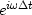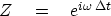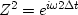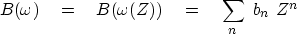Next: Unit circle Up: Convolution and Spectra Previous: Fourier sum

# FOURIER AND Z-TRANSFORM

The frequency function of a pulse at timeis.The factoroccurs so often in applied work that it has a name:(20)
With this Z, the pulse at time tn is compactly represented as Zn. The variable Z makes Fourier transforms look like polynomials, the subject of a literature called Z-transforms.'' The Z-transform is a variant form of the Fourier transform that is particularly useful for time-discretized (sampled) functions.

From the definition (20), we have,,etc. Using these equivalencies, equation (19) becomes(21)Next: Unit circle Up: Convolution and Spectra Previous: Fourier sum
Stanford Exploration Project
10/21/1998